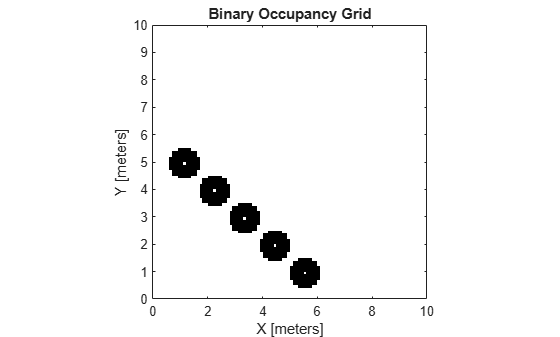Documentation

# inflate

Inflate each occupied grid location

## Syntax

``inflate(map,radius)``
``inflate(map,gridradius,'grid')``

## Description

example

````inflate(map,radius)` inflates each occupied position of the `map` by the radius given in meters. `radius` is rounded up to the nearest cell equivalent based on the resolution of the map. Every cell within the radius is set to `true` (`1`).```
````inflate(map,gridradius,'grid')` inflates each occupied position by the radius given in number of cells.```

## Examples

collapse all

Create a 10m x 10m empty map.

`map = binaryOccupancyMap(10,10,10);`

Set occupancy of world locations and show map.

```x = [1.2; 2.3; 3.4; 4.5; 5.6]; y = [5.0; 4.0; 3.0; 2.0; 1.0]; setOccupancy(map, [x y], ones(5,1)) figure show(map)```Inflate occupied locations by a given radius.

```inflate(map, 0.5) figure show(map)```Get grid locations from world locations.

`ij = world2grid(map, [x y]);`

Set grid locations to free locations.

```setOccupancy(map, ij, zeros(5,1), 'grid') figure show(map)```## Input Arguments

collapse all

Map representation, specified as a `binaryOccupancyMap` object. This object represents the environment of the robot. The object contains a matrix grid with binary values indicating obstacles as `true` (`1`) and free locations as `false` (`0`).

Dimension that defines how much to inflate occupied locations, specified as a scalar. `radius` is rounded up to the nearest cell value.

Data Types: `double`

Dimension that defines how much to inflate occupied locations, specified as a positive scalar. `gridradius` is the number of cells to inflate the occupied locations.

Data Types: `double`